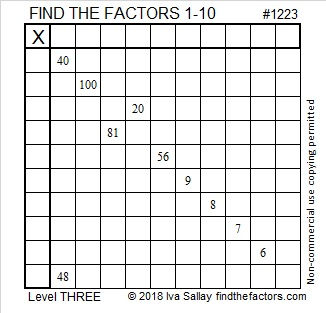# 1223 and Level 3

If you’ve been too anxious to try solving a level 3 puzzle in the past, you have no excuse for not trying this one. This might be the easiest level 3 puzzle I’ve ever published. Just write the factors for 40 and 48 in the proper cells, then work your way down the puzzle writing only numbers from 1 to 10 in the first column and the top row. Seriously, you can do this one!Print the puzzles or type the solution in this excel file: 10-factors-1221-1231

Now I’ll write a little about the number 1223:

• 1223 is a prime number.
• Prime factorization: 1223 is prime.
• The exponent of prime number 1223 is 1. Adding 1 to that exponent we get (1 + 1) = 2. Therefore 1223 has exactly 2 factors.
• Factors of 1223: 1, 1223
• Factor pairs: 1223 = 1 × 1223
• 1223 has no square factors that allow its square root to be simplified. √1223 ≈ 34.97142

How do we know that 1223 is a prime number? If 1223 were not a prime number, then it would be divisible by at least one prime number less than or equal to √1223 ≈ 34.97. Since 1223 cannot be divided evenly by 2, 3, 5, 7, 11, 13, 17, 19, 23, 29 or 31, we know that 1223 is a prime number.1223 is the sum of the twenty-one prime numbers from 17 to 103.

This site uses Akismet to reduce spam. Learn how your comment data is processed.